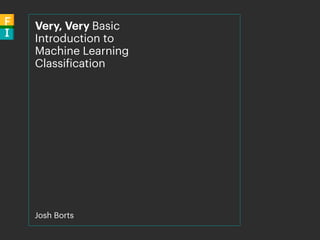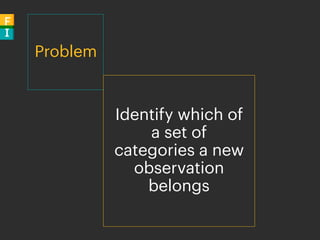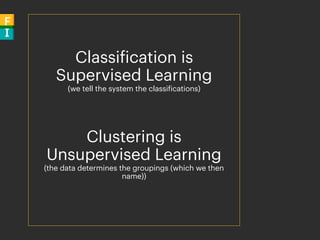Successfully reported this slideshow.

# Introduction to Machine Learning Classifiers×

# Introduction to Machine Learning Classifiers

You will learn the basic concepts of machine learning classification and will be introduced to some different algorithms that can be used. This is from a very high level and will not be getting into the nitty-gritty details.

You will learn the basic concepts of machine learning classification and will be introduced to some different algorithms that can be used. This is from a very high level and will not be getting into the nitty-gritty details.

### Introduction to Machine Learning Classifiers

1. 1. Very, Very Basic Introduction to Machine Learning Classification Josh Borts
2. 2. Problem Identify which of a set of categories a new observation belongs
3. 3. Classification is Supervised Learning (we tell the system the classifications) Clustering is Unsupervised Learning (the data determines the groupings (which we then name))
4. 4. Examples
5. 5. Handwriting Recognition / OCR
6. 6. Spam Filters
7. 7. Blood Type Identification
8. 8. Automatic Document Classification
9. 9. Face Recognition
10. 10. SHAZAM!!
11. 11. Other Examples Credit Scoring Text Sentiment Extraction Cohort Assignment Gesture Recognition
12. 12. Observations an Observation can be described by a fixed set of quantifiable properties called Explanatory Variables or Features
13. 13. For example, a Doctor visits could result in the following Features: • Weight • Male/Female • Age • White Cell Count • Mental State (bad, neutral, good, great) • Blood Pressure • etc
14. 14. Text Documents will have a set of Features that defines the number of occurrences of each Word or n-gram in the corpus of documents
15. 15. Classifier a Machine Learning Algorithm or Mathematical Function that maps input data to a category is known as a Classifier Examples: • Linear Classifiers • Quadratic Classifiers • Support Vector Machines • K-Nearest Neighbours • Neural Networks • Decision Trees
16. 16. Most algorithms are best applied to Binary Classification. If you want to have multiple classes (tags) then use multiple Binary Classifiers instead
17. 17. Training A Classifier has a set of variables that need to set (trained). Diﬀerent classifiers have diﬀerent algorithms to optimize this process
18. 18. Overfitting Danger!! The model fits only the data in was trained on. New data is completely foreign
19. 19. Among competing hypotheses, the one with the fewest assumptions should be selected
20. 20. Split the data into In-Sample (training) and Out-Of-Sample (test)
21. 21. How do we Evaluate Classifier Performance?
22. 22. Of course there are many ways we can define Best Performance… Accuracy Sensitivity Specifity F1 Score Likelihood Cumulative Gain Mean Reciprocal Rank Average Precision
23. 23. Algorithms
24. 24. k-Nearest Neighbor Cousin of k-Means Clustering Algorithm: 1) In feature space, find the k closest neighbors (often using Euclidean distance (straight line geometry)) 2) Assign the majority class from those neighbors
25. 25. Decision Tress Can generate multiple decision trees to improve accuracy (Random Forest) Can be learned by consecutively splitting the data on an attribute pair using Recursive Partitioning
26. 26. New York & San Fran housing by Elevation and Price
27. 27. Linear Classifier
28. 28. Linear Combination of the Feature Vector and a Weight Vector. Can think of it as splitting a high-dimensional input space with a hyperplane
29. 29. Often the fastest classifier, especially when feature space is sparse or large number of dimensions
30. 30. Determining the Weight Vector Can either use Generative or Discriminative models to determine the Weight Vector
31. 31. Generative models attempt to model the conditional probability function of an Observation Vector given a Classification. Examples include: • LDA (Gaussian density) • Naive Bayes Classifier (Multinomial Bernoulli events)
32. 32. Examples include: • Logistic Regression (maximum likelihood estimation assuming training set was generated by a binomial model) • Support Vector Machine (attempts to maximize the margin between the decision hyperplane and the examples in the training set) Discriminative models attempt to maximize the quality of the output on a training set through an optimization algorithm.
33. 33. Neural Network Not going to get into the details, this time….
34. 34. Functional Imperative functionalimperative.com (647) 405-8994 @func_i